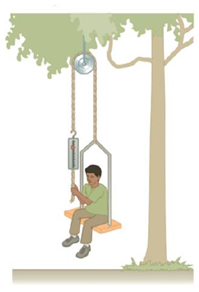Chapter 4, Problem 88AP

Chapter
Section
Textbook Problem

An inventive child wants to reach an apple in a tree without climbing the tree. Sitting in a chair connected to a rope that passes over a frictionless pulley (Fig. P4.88), the child pulls on the loose end of the rope with such a force that the spring scale reads 250 N. The child’s true weight is 320- N, and the chair weighs 160 N. The child’s feet are not touching the ground. (a) Show that the acceleration of the system is upward, and find its magnitude. (b) Find the force the child exerts on the chair.Figure P4.88

(a)

To determine
The acceleration of the system.

Explanation

Given info: Weight of the child is 320 N. Weight of the chair is 160 N. Reading on the spring scale is 250 N.

The free body diagram is given below.

• T is the tension.
• Wt is the total weight.
• a is the acceleration.
• N is the normal force.

Total weight is,

Wt=(320N)+(160N)=480N

From the diagram,

2TWt=(Wtg)a

On Re-arranging, the acceleration is,

a=g(2TWt1)

Substitute 9

(b)

To determine
The force exerted by the child on the chair.

Still sussing out bartleby?

Check out a sample textbook solution.

See a sample solution

The Solution to Your Study Problems

Bartleby provides explanations to thousands of textbook problems written by our experts, many with advanced degrees!

Get Started